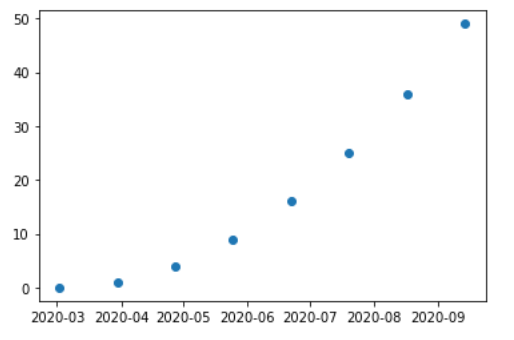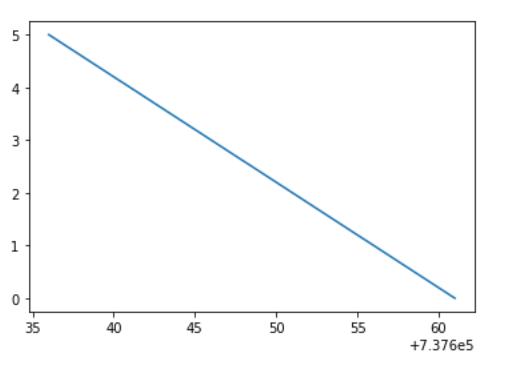# Matplotlib.dates.drange() in Python

Matplotlib is an amazing visualization library in Python for 2D plots of arrays. Matplotlib is a multi-platform data visualization library built on NumPy arrays and designed to work with the broader SciPy stack.

## matplotlib.dates.drange()

The `matplotlib.dates.drange()` function returns a sequence of equally spaced Matplotlib dates. The date range starts from ‘dstart’ and go up to, but not including ‘dend’. The space between each date is called delta.

Syntax: matplotlib.dates.drange(dstart, dend, delta)

Parameters:

1. dstart: Starting point of the date range and is a python’s datetime date.
2. dend: Ending point of the date range and is a python’s datetime date.
3. delta: It represents the spacing between each dates and belongs to python’s datetime.timedelta.

Returns: It returns a numpy array which is a list of floats representing Matplotlib dates.

Example 1:

 `import` `datetime ` `import` `matplotlib.pyplot as plt ` `from` `matplotlib.dates ``import` `DayLocator, HourLocator, DateFormatter, drange ` `import` `numpy as np ` ` `  ` `  `date_1 ``=` `datetime.datetime( ``2020``, ``3``, ``2``) ` `date_2 ``=` `datetime.datetime( ``2020``, ``10``, ``10``) ` ` `  `time_delta ``=` `datetime.timedelta(days ``=` `28``) ` `dates ``=` `drange(date_1, date_2, time_delta) ` ` `  `y_axis ``=` `np.arange( ``len``(dates) ) ` ` `  `fig, ax ``=` `plt.subplots() ` `ax.plot_date(dates, y_axis ``*` `y_axis) ` ` `  `ax.xaxis.set_major_formatter( DateFormatter(``'% Y-% m'``) ) ` ` `  `plt.show() `

Output:Example 2:

 `import` `datetime ` `import` `matplotlib.pyplot as plt ` `import` `matplotlib.dates as mdates ` ` `  `date ``=` `[datetime.datetime(``2020``, ``8``, ``24``, ``0``, ``0``), ` `        ``datetime.datetime(``2020``, ``8``, ``23``, ``0``, ``0``),  ` `        ``datetime.datetime(``2020``, ``8``, ``22``, ``0``, ``0``),  ` `        ``datetime.datetime(``2020``, ``8``, ``21``, ``0``, ``0``),  ` `        ``datetime.datetime(``2020``, ``8``, ``18``, ``0``, ``0``), ` `        ``datetime.datetime(``2020``, ``8``, ``17``, ``0``, ``0``), ` `        ``datetime.datetime(``2020``, ``8``, ``16``, ``0``, ``0``), ` `        ``datetime.datetime(``2020``, ``8``, ``15``, ``0``, ``0``), ` `        ``datetime.datetime(``2020``, ``8``, ``14``, ``0``, ``0``), ` `        ``datetime.datetime(``2020``, ``8``, ``11``, ``0``, ``0``), ` `        ``datetime.datetime(``2020``, ``8``, ``10``, ``0``, ``0``),  ` `        ``datetime.datetime(``2020``, ``8``, ``9``, ``0``, ``0``), ` `        ``datetime.datetime(``2020``, ``8``, ``8``, ``0``, ``0``), ` `        ``datetime.datetime(``2020``, ``8``, ``7``, ``0``, ``0``), ` `        ``datetime.datetime(``2020``, ``8``, ``4``, ``0``, ``0``), ` `        ``datetime.datetime(``2020``, ``8``, ``3``, ``0``, ``0``), ` `        ``datetime.datetime(``2020``, ``8``, ``2``, ``0``, ``0``), ` `        ``datetime.datetime(``2020``, ``8``, ``1``, ``0``, ``0``)] ` ` `  `# is a datetime.datetime object  ` `# according to type ` `start_date ``=` `date[``0``] ` ` `  `# is a datetime.datetime object according  ` `# to type     ` `end_date ``=` `date[``-``1``] ` `delta ``=` `datetime.timedelta(days ``=` `5``) ` ` `  `# the drange function ` `dates ``=` `mdates.drange(start_date, end_date, ``-``delta) ` `y_data ``=` `range``(``len``(dates)) ` ` `  `plt.plot(dates, y_data) `

Output:My Personal Notes arrow_drop_upCheck out this Author's contributed articles.

If you like GeeksforGeeks and would like to contribute, you can also write an article using contribute.geeksforgeeks.org or mail your article to contribute@geeksforgeeks.org. See your article appearing on the GeeksforGeeks main page and help other Geeks.

Please Improve this article if you find anything incorrect by clicking on the "Improve Article" button below.

Article Tags :

Be the First to upvote.

Please write to us at contribute@geeksforgeeks.org to report any issue with the above content.